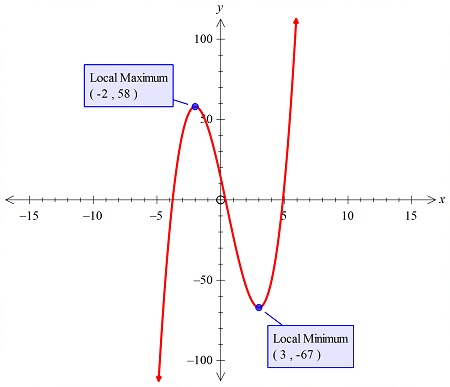# Find all relative extrema of the following function and show it in a table: F(x)=10-3x^2-36x+2x^3+4

## Question:

Find all relative extrema of the following function and show it in a table: {eq}F(x)=10-3x^2-36x+2x^3+4{/eq}

## Minima & Maxima:

If the maxima and the minima are solved with one of the two approaches, then whatever method we used, we get the same result. For the graphical method, we plot the points of extremes in the same plot as that of the curve.

The graph of the function is shown and the relative extremes are also shown below:Bow the local minima is at:

{eq}(3,-67) {/eq}

and local extrema is at:

{eq}(-2,58) {/eq}